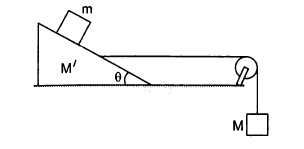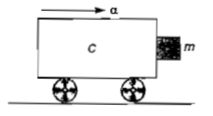You’ve reached the end of your free Videos limit.
#34-Failure-of-Newton's-Laws
(Physics) > Laws of Motion
Unable to watch the video, please try another server
Related Practice Questions :

The value of M of the hanging block is in the figure, which will prevent the smaller block (m=1 kg) from slipping over the triangular block. All the surfaces are smooth and string and pulley are ideal. (Given: M'=4 kg and $\theta ={37}^{0}$)1. 12 kg

2. 15 kg

3. 10 kg

4. 4 kg

High Yielding Test Series + Question Bank - NEET 2020

Difficulty Level:

A plumb line is suspended from a ceiling of a car moving with horizontal acceleration of a. What will be the angle of inclination with vertical

(1) tan–1(a/g)

(2) tan–1(g/a)

(3) cos–1(a/g)

(4) cos–1(g/a)

High Yielding Test Series + Question Bank - NEET 2020

Difficulty Level:

A block is kept on a frictionless inclined surface with angle of inclination 'α'. The incline is given an acceleration 'a' to keep the block stationary. Then a is equal to

(1) g

(2) g tan α

(3) g / tan α

(4) g cosec α

High Yielding Test Series + Question Bank - NEET 2020

Difficulty Level:

On the horizontal surface of a truck (μ = 0.6), a block of mass 1 kg is placed. If the truck is accelerating at the rate of 5m/sec2 then frictional force on the block will be

(1) 5 N

(2) 6 N

(3) 5.88 N

(4) 8 N

High Yielding Test Series + Question Bank - NEET 2020

Difficulty Level:

A block of mass m is in contact with the cart C as shown in the figure.The coefficient of static friction between the block and the cart is $\mu .$The acceleration $\alpha$ of the cart that will prevent the block from falling satisfies

1. $\alpha >\frac{mg}{\mu }$                                       2. $\alpha >\frac{g}{\mu m}$

3. $\alpha \ge \frac{g}{\mu }$                                          4. $\alpha <\frac{g}{\mu }$